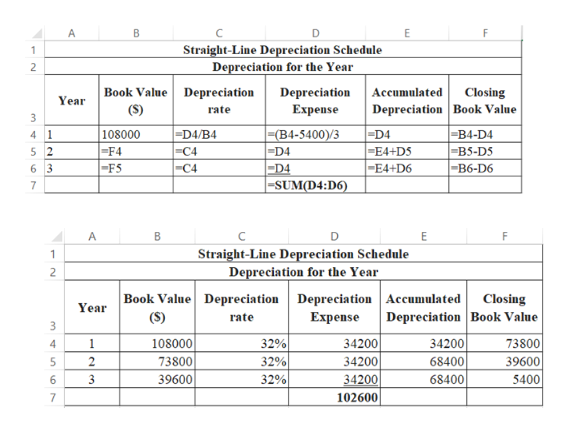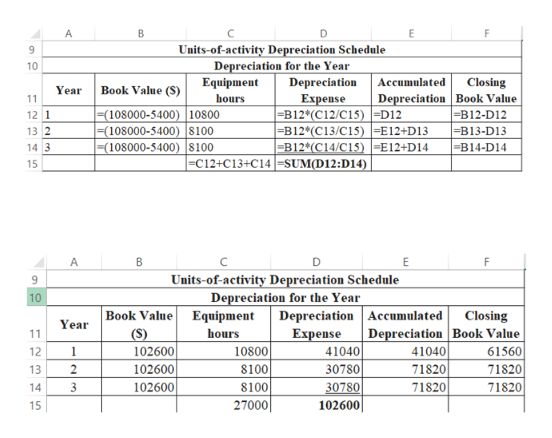# Comparing three depreciation methodsDexter Industries purchased packaging equipment on January 8 for \$108,000. The equipment was expected to have a useful life of three years, or 27,000 operating hours, and a residual value of \$5,400. The equipment was used for 10,800 hours during Year 1, 8,100 hours in Year 2, and 8,100 hours in Year 3.Required:1.  Determine the amount of depreciation expense for the three years ending December 31, by (a) the straight-line method, (b) the units-of-activity method, and (c) the double-declining-balance method. Also determine the total depreciation expense for the three years by each method. Round the final answers for each year to the nearest whole dollar.

Question
23 views

Comparing three depreciation methods

Dexter Industries purchased packaging equipment on January 8 for \$108,000. The equipment was expected to have a useful life of three years, or 27,000 operating hours, and a residual value of \$5,400. The equipment was used for 10,800 hours during Year 1, 8,100 hours in Year 2, and 8,100 hours in Year 3.

Required:

1.  Determine the amount of depreciation expense for the three years ending December 31, by (a) the straight-line method, (b) the units-of-activity method, and (c) the double-declining-balance method. Also determine the total depreciation expense for the three years by each method. Round the final answers for each year to the nearest whole dollar.

check_circle

Step 1

(a) Calculation of depreciation under straight-line method:

Results of the excel sheet is as follows:help_outlineImage TranscriptioncloseStraight-Line Depreciation Schedule Depreciation for the Year Book Value Depreciation (S) Depreciation Accumulated Closing Year Depreciation Book Value Expense rate 3 |=(B4-5400)/3 -D4 -D4 =SUM(D4:D6) -D4/B4 =C4 -C4 -D4 |-E4+DS -E4+D6 108000 -B4-D4 4 -F4 -F5 -B5-D5 -B6-D6 6 3 Straight-Line Depreciation Schedule Depreciation for the Year Book Value Depreciation Depreciation Accumulated (S) Closing Depreciation Book Value Year Expense rate 108000 73800 39600 32% 73800 34200 34200 1. 34200 68400 32% 39600 68400 3 32% 34200 5400 102600 fullscreen
Step 2

(b) Calculation of depreciation under the units-of-activity method:

Resu...help_outlineImage TranscriptioncloseA Units-of-activity Depreciation Schedule Depreciation for the Year Equipment 9. 10 Depreciation Accumulated Closing Depreciation Book Value |=B12-D12 Book Value (S) Year hours Expense =B12*(C12/C15) =D12 |=B12*(C13/C15) =E12+D13 -B12*(C14/C15) -E12+D14 11 =(108000-5400) 10800 =(108000-5400) 8100 (108000-5400) 8100 12 1 13 2 =B13-D13 =B14-D14 14 3 |=C12+C13+C14 =SUM(D12:D14) 15 B Units-of-activity Depreciation Schedule Depreciation for the Year 10 Book Value Equipment (S) 102600 Depreciation Accumulated Expense 41040 Closing Year Depreciation Book Value hours 11 10800 8100 8100 27000 12 1 41040 61560 102600 102600 30780 71820 71820 13 30780 71820 71820 14 102600| 15 fullscreen

### Want to see the full answer?

See Solution

#### Want to see this answer and more?

Solutions are written by subject experts who are available 24/7. Questions are typically answered within 1 hour.*

See Solution
*Response times may vary by subject and question.
Tagged in